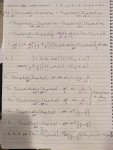3D Heat Equation Using Cylindrical Coordinate System

Amelia_g

New member
I am looking at constructing a set of equations to describe the temperature of a 3D cylinder. I have found this paper: http://csbe-scgab.ca/docs/journal/32/32_2_315_ocr.pdf which very closely describes the situation I am trying to model, however, I am having trouble understanding the derivation of Equation 4 and 5 (pg. 316). The paper begins with an equation for the interior nodes and applies boundary conditions to generate equations for the nodes along the edge and center of the cylinder. Equation 5 is the equation specific to the nodes along the external, vertical edge of the cylinder. It is just the coefficient for (T(m , n+1, l)+T(m, n-1, l)) that I cannot understand.

This is my working:(Note that in my working: Fo = Fourier's Number = 1/CC and Bi = Biot's Number = h.(del r) / k).

Equation 4 is for the nodes along the central axis and I am not sure why it has been expressed as a summation of 10 nodes, when there appears to be only five conduction faces for the central element, as shown in Figure 1?

Anyone able to help with the steps I am missing would be much appreciated!

Last edited: